# Geometric Tools: Description And Uses

Geometric tools are the instruments used to draw different types of geometric shapes. In Maths, geometry is the most crucial topic, where we learn about the shapes of objects. To draw these shapes, there are different types of tools with different names used while solving problems based on geometry. We come across a number of shapes in our day to day life. You are already aware of a couple of them such as triangles, squares, hexagons, circles, parallelograms and so on. Different figures have characteristic properties such as length, breadth, diameter, etc. which set them apart from one another and are parameters to produce the figures on paper. Practical geometry or Euclidean geometry is the most pragmatic branch of geometry that deals with the construction of different geometrical figures using geometric instruments such as rulers, compasses and protractors. In this article, we are going to discuss important Maths tools which are used in Geometry for the construction of different shapes with a complete explanation.

## Geometric Tools and Their Uses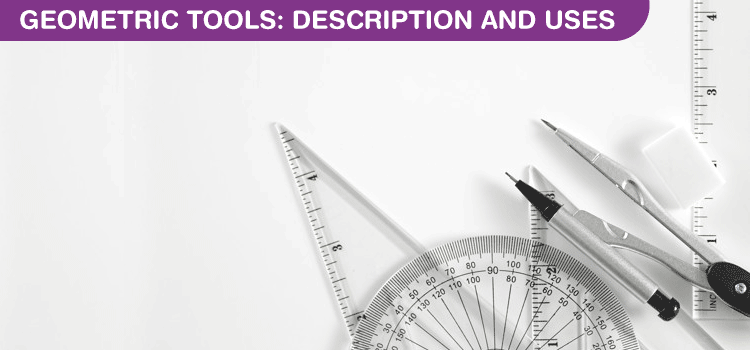The early geometers studied figures such as points, lines and angles which required the use of rulers and compasses only. With the advent of more geometric designs, other geometric tools were invented. Some of the most commonly used geometric tools are:

Now, let us discuss the different Maths tools with their description and uses in detail.

## Geometric Tool – Ruler

Also known as the straightedge, a ruler is used to construct straight lines and measure the lengths of a line segment. A ruler is also known as the line gauge, is a mathematical tool used in Geometry as well as in many Engineering applications.  It is a straight edge initially used only for drawing straight lines. It can also be used to measure the distance of the object. However, for our convenience, it is graduated into centimetres and millimetres on one side and into inches on the other side. It is used to measure the lengths in both the metric units as well as in customary units. The interval or the marks mentioned in the ruler is called the “Hash Marks”.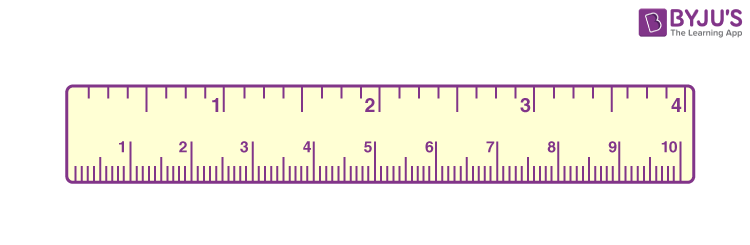How to Measure Objects Using a Ruler?

If we want to measure the length of the object using a ruler, we need to place the zero hash mark of the ruler on the one end of the object. Now, align the object along the edge of the ruler. Read the measurement on the hash mark of the ruler, in which the other side of the object ends. The distance between the zero hash mark and the final reading hashmark on the ruler is called the length of the object.

## Geometric Tool – Compass

It is a ‘V’-shaped tool that holds a pencil on one side of the ‘V’ and a pointer on the other side. The distance between the pencil and the pointer is adjustable. It is used to trace arcs, circles and angles. It is also used to mark equal lengths. Compass is one of the mathematical tools which is used to draw a geometrical figure, such as a circle. It is also used in the intersection of line segments and tools, which is used to intersect the line segment and helps to find the midpoint of the shapes.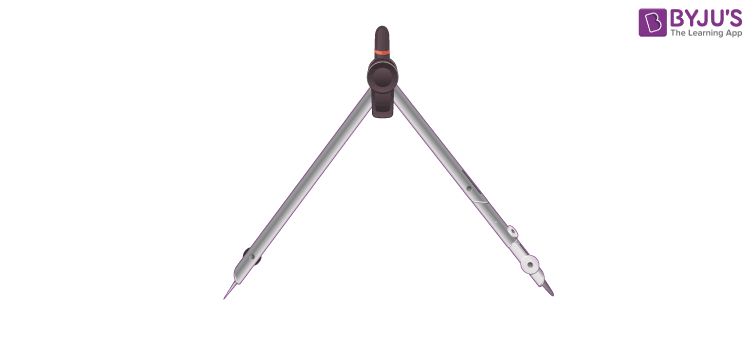## Geometric Tool – Protractor

A protractor in Maths is used to measure angles. It is a semi-circular disc used to draw and measure different types of angles. It is graduated from 0 to 180 degrees and can be directly used to measure any angle within its range. It has two sets of markings, 0 to 180 degrees from left to right and vice versa. The inner and the outer reading of the protractor supplements each other. It means that if we add inner and outer reading, it adds up to 180 degrees.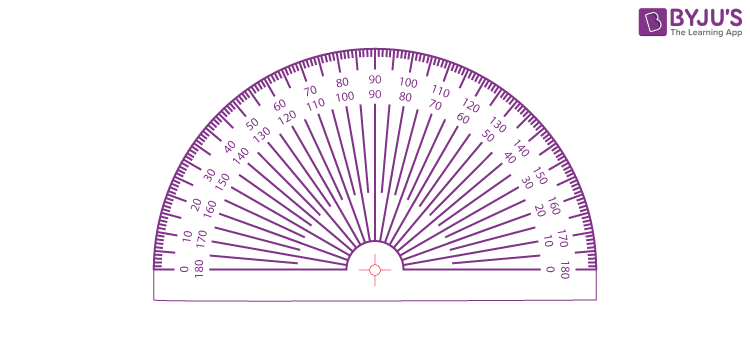While measuring an angle using the protractor, we need to follow the steps given below:

• If the angle to be measured at the left side of the protractor, we have to use the outer reading provided in the protractor.
• If the angle to be measured at the right side of the protractor, we have to use the inner reading provided in the protractor.

## Geometric Tool – Divider

It looks similar to the compasses with its ‘V’-shaped structure. However, it has pointers on both ends of the ‘V’. The distance between them is adjustable and it is used to measure and compare lengths.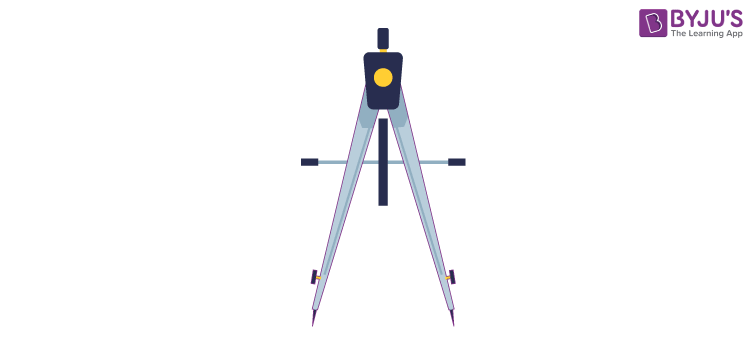The term divider and compass are often used to mark the distance or mark divisions. The major difference between the compass and divider is that the compass is defined as a drafting instrument, in which one sharp point is positioned at centre and the other point has a pen or pencil to describe the circle or other functionality. Whereas the divider has two sharp points, in which one sharp point used as a centre, and the other sharp point is used for marking.

## Geometric Tool – Set-Squares

Set squares are also one of the Maths tool, which is found commonly in geometry box. These are the triangular pieces of plastic with some portion between them removed. There are two kinds of set squares available in the market. One has an angle of 45 degrees and the other has 30-60 degrees. The 45 degree set square has an angle of 90 degrees, and also 30/60 degree set square has a right angle. The 45 degrees set square is used to draw vertical lines. With the help of set squares, we can draw parallel lines, perpendicular lines, make some standard angles, and so on.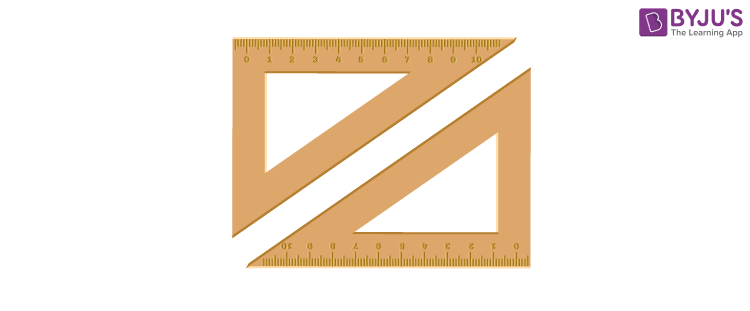## Video Lesson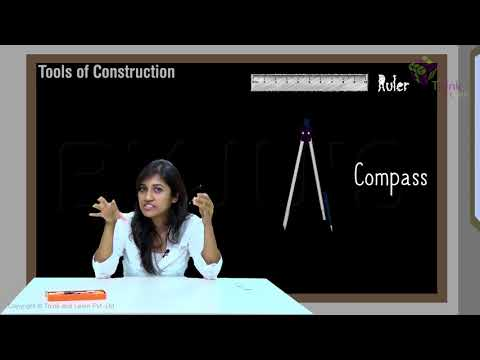## Frequently Asked Questions on Geometric Tools

Q1

### What are Geometric Tools in Maths?

Geometric tools are those instruments that are used to construct different types of geometric shapes and figures. In Euclidean geometry, all the shapes, lines, and angles are drawn using geometric tools.

Q2

### What are the 2 Most Important Euclidean Tools Used in Geometric Construction?

The two most important Euclidean tools used by early Greeks to construct different geometrical shapes and angles were a compass and a straightedge. Using these two tools, almost any shape can be constructed. Later, rulers and protectors were invented which made construction easier.

Q3

### What are the Most Common Instruments in a Geometry Box?

The most common tools in a student geometric box are:

• Compass
• Ruler
• Protractor
• Divider
• Set-squares
Q4

### What is the use of a Ruler in Geometry?

In Geometry, a ruler is used to draw straight lines. Rulers are also used to measure the length of the object.

Q5

### Why do we use Protractor in Maths?

A protractor is used to measure the angles. The protractor is graduated from 0 degree to 180 degrees, which helps to measure the angle value. It has two sets of markings from 0-180 degree from left to right as well as from right to left. The inner and outer angles will add up to 180 degrees.

Quiz on Geometric tools